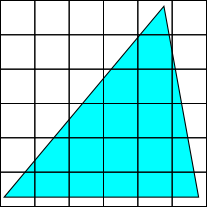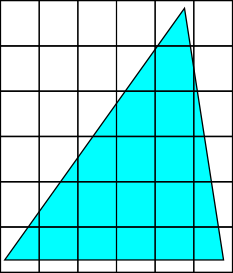#### You may also like### Building Tetrahedra

Can you make a tetrahedron whose faces all have the same perimeter?A 1 metre cube has one face on the ground and one face against a wall. A 4 metre ladder leans against the wall and just touches the cube. How high is the top of the ladder above the ground?Four rods are hinged at their ends to form a convex quadrilateral. Investigate the different shapes that the quadrilateral can take. Be patient this problem may be slow to load.

# Growing Triangle

##### Age 14 to 16 ShortChallenge Level
Considering triangles with area 100 square units
If the area of the original triangle is 100 square units, then each square unit represents 1%. So by enlarging the base and height of the triangle and finding the new area, it will be clear what the percentage increase has been.

Both of the triangles shown below have area 100 square units, since $\frac{1}{2}\times10\times20=\frac{1}{2}\times8\times25=100$.Increasing the base of each triangle by 20%
• 20% of 20 is 4, so the new base is 24
• 20% of 8 is 1.6, so the new base is 9.6

Increasing the height of each triangle by 30%
• 30% of 10 is 3, so the new height is 13
• 30% of 25 is 7.5, so the new height is 32.5

This is shown below.The area of the left hand triangle is $\frac{1}{2}\times24\times13=156$ square units.

The area of the right hand triangle is $\frac{1}{2}\times9.6\times32.5=156$ too (and they must be the same, or the question wouldn't make sense).
In both cases, the area has increased by 56 units, which is 56% of 100 units. So the area has increased by 56%.

Enlarging a unit by 20% and 30%
Imagine that the triangle is on a squared grid, then the area of the triangle is the number of squares (including fractions of squares) that fit inside it.Then when the triangle has been enlarged, the whole grid could have been enlarged - so the triangle still contains the same number of 'squares', but the squares have changed shape.Now, instead of being 1 by 1 squares with area 1, the squares have become rectangles, because their bases have been increased by 20% and their heights have been increased by 30%.
1 increased by 20% is 1.2, and 1 increased by 30% is 1.3, so the rectangles are 1.2 by 1.3.$1.2\times1.3=1.56$. So the area of each square has been increased by 0.56, which is the same as 56% of its original area of 1. So since each square has increased in area by 56%, the area of the triangle has also been increased by 56%.

Using length and width scale factors
Increasing the base by 20% is a horizontal stretch, and so the area will be increased by 20%, to 120% of its original area.

Increasing the height by 30% is a vertical stretch, and so the new area will be increased by 30%. 30% of 120% is 36%, so the new area will be 156% of the original area.

So the area has increased by 56%.

Using algebra for the base and height
Suppose the triangle had base $b$ and height $h$. Then its area was $\frac{1}{2}b\times h$.

When the base is increased by 20%, the new base will be $1.2b$. When the height is increased by 30%, the new height will be $1.3h$.

So the new area will be $\frac{1}{2}\times1.2b\times1.3h=\frac{1}{2}\times b\times h\times1.2\times1.3$.

That is the same as the old area multiplied by $1.2\times1.3=1.56$, so the area has been multiplied by 1.56, which corresponds to an increase of 56%.
You can find more short problems, arranged by curriculum topic, in our short problems collection.